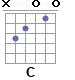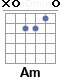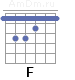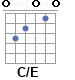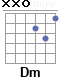Уважаемые пользователи, в данный момент ведутся работы по настройке мобильной версии сайта и поэтому часть функций не работает или работает не корректно.

# Beatles - Let It Be, аккорды

• 370,349
• 786
• 30 нояб. 1999
Прокрутка
Тон-сть
Шрифт
```       C              G                 Am          F
When I find myself in times of trouble, Mother Mary comes to me
C             G  F  C/E Dm C
Speaking words of wisdom, let it be
C              G                 Am          F
And in my hour of darkness, She is standing right in front of me
C             G  F  C/E Dm C
Speaking words of wisdom, Let it be

Chorus:
Am         G          F          C
Let it be, let it be, let it be, let it be
C                G              F  C/E Dm C
Whisper words of wisdom, let it be

C              G                 Am          F
And when the broken hearted people, Living in the world agree
C             G  F  C/E Dm C
There will be an answer, let it be
C              G                 Am          F
But though they may be parted, There is still a chance
that they may see
C             G  F  C/E Dm C
There will be an answer, let it be

Chorus:
Am         G          F          C
Let it be, let it be, let it be, let it be
C                G              F  C/E Dm C
There will be an answer, let it be
Am         G          F          C
Let it be, let it be, let it be, let it be
C                G              F  C/E Dm C
Whisper words of wisdom, let it be

Am         G          F          C
Let it be, let it be, let it be, let it be
C                G              F  C/E Dm C
Whisper words of wisdom, let it be
C              G                 Am          F
And when the night is cloudy, there is still a light that shines on me
C                G              F  C/E Dm C
Shine on till tomorrow, let it be
C              G                 Am          F
I wake up to the sound of music, Mother Mary comes to me
C                G              F  C/E Dm C
Speaking words of wisdom, let it be

Chorus:
Am         G          F          C
Let it be, let it be, let it be, let it be
C                G              F  C/E Dm C
There will be an answer, let it be
Am         G          F          C
Let it be, let it be, let it be, let it be
C                G              F  C/E Dm C
Whisper words of wisdom, let it be```

### Аппликатуры аккордов